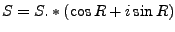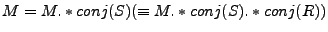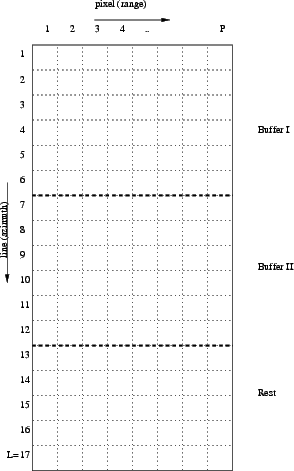Next: COMPREFPHA Up: INTERFERO Previous: Output Description   Contents

# Implementation

The following is computed (in buffers).

1. Read in (buffer of) complex master and slave image (M and S).

2. If there is a reference phase R (real values), evaluate it at the master grid (buffer), and then subtract it (in a complex way) from slave S and store it (in S). (matlab like notation, pointwise multiplication notated by .*)(W4)

3. Compute complex interferogram and store it in M.(W5)

4. Multilook complex interferogram if requested. Do not divide (scale) multilooked interferogram.

5. Write this buffer to disk and start next one.See Figure 23.2 for an explanation of the use of buffers in the implementation. In this example, the number of lines equals 17. The number of pixels equals P. Suppose multilook factor in line direction mL=3 and in pixel direction mP=3. Furthermore, after computing the available memory, the number of lines of one buffer (BL) is maximum 7. BL is set to a multiple of mL, BL=6.

Now the number of fully filled buffers NB = 17/6 = 2. The number of lines left in the last buffer equals 17We can compute something in this last buffer only for the first three lines, so the last buffer is 3 lines long.

The first buffer is read (master and slave image), and computations are performed. These results are written to disk. Then next buffer, etc. If buffers==3 then resize the matrices for the computations.

The number of lines of the total result are L/mL and P/mP (floored).Next: COMPREFPHA Up: INTERFERO Previous: Output Description   Contents
Leijen 2009-04-14